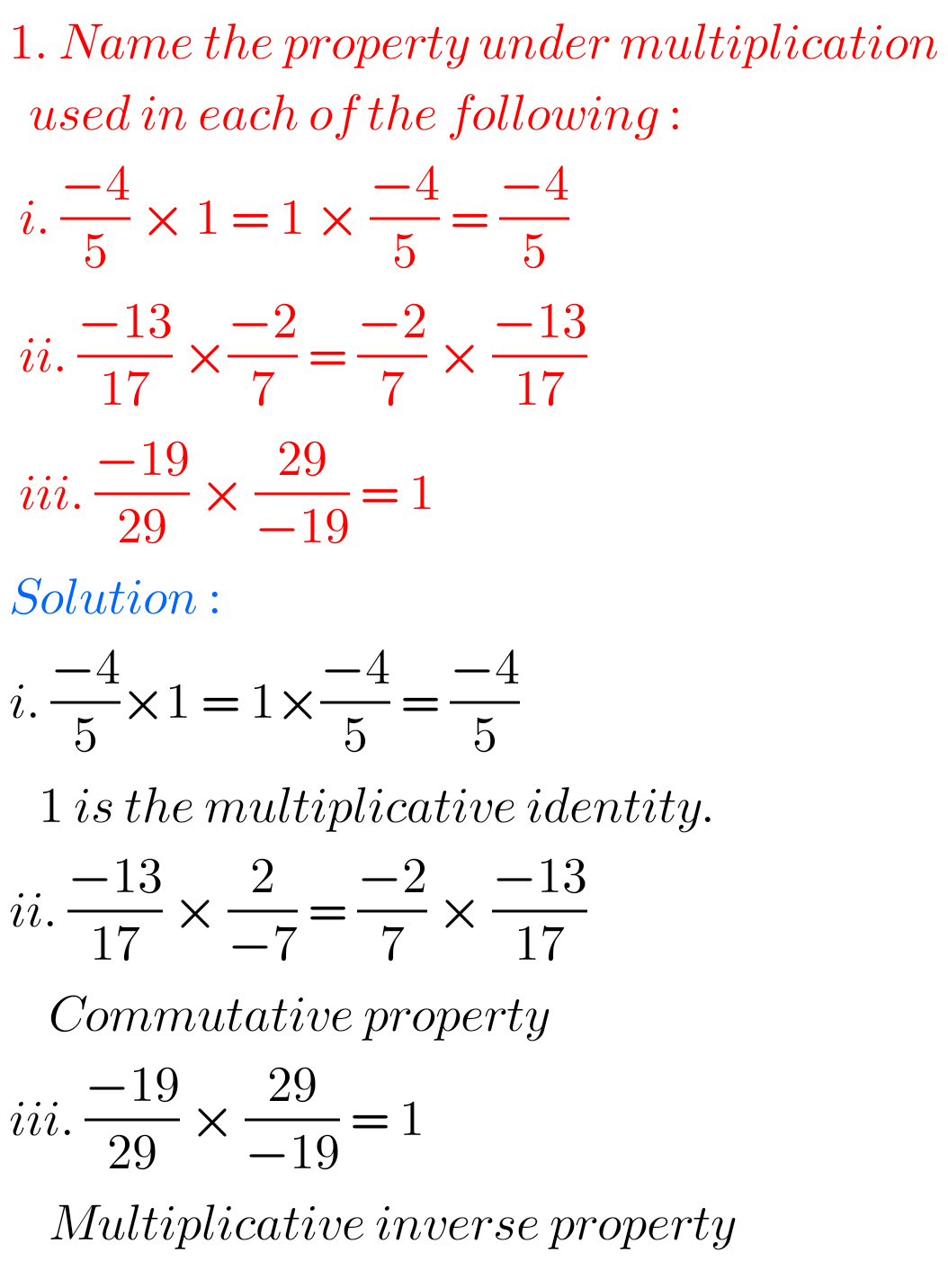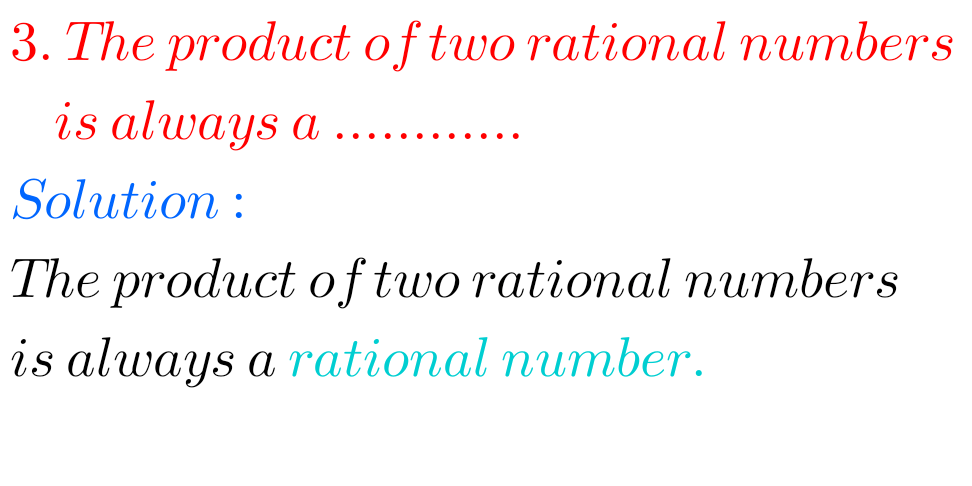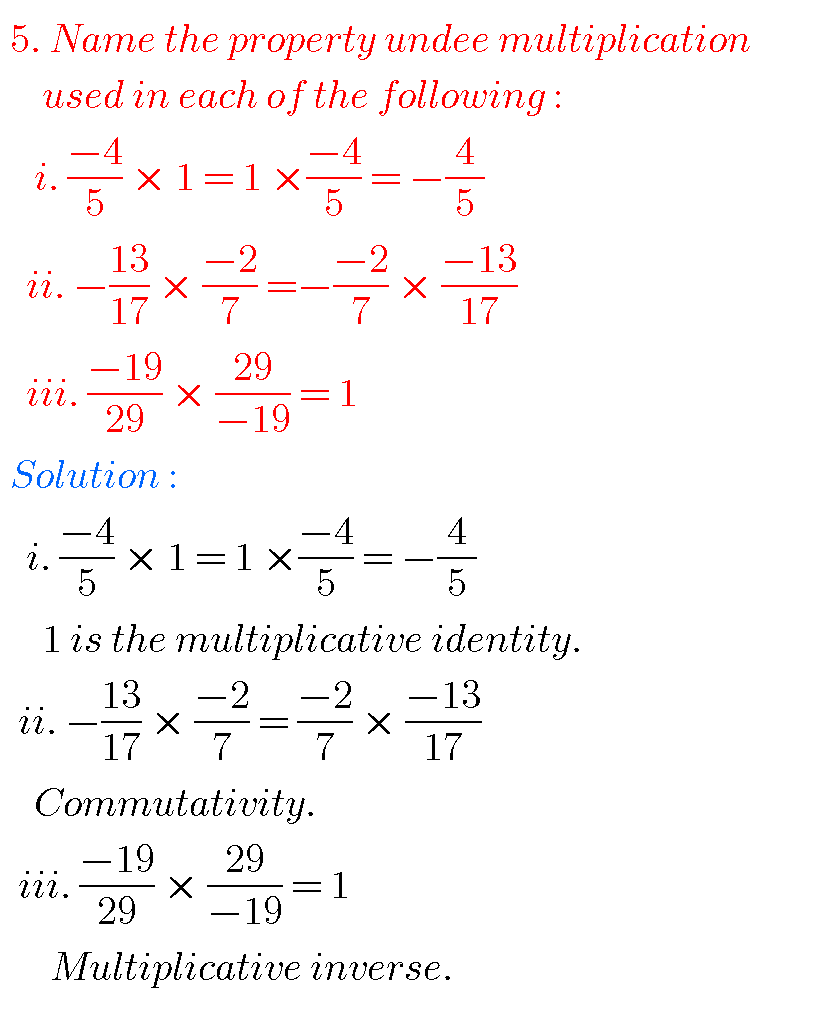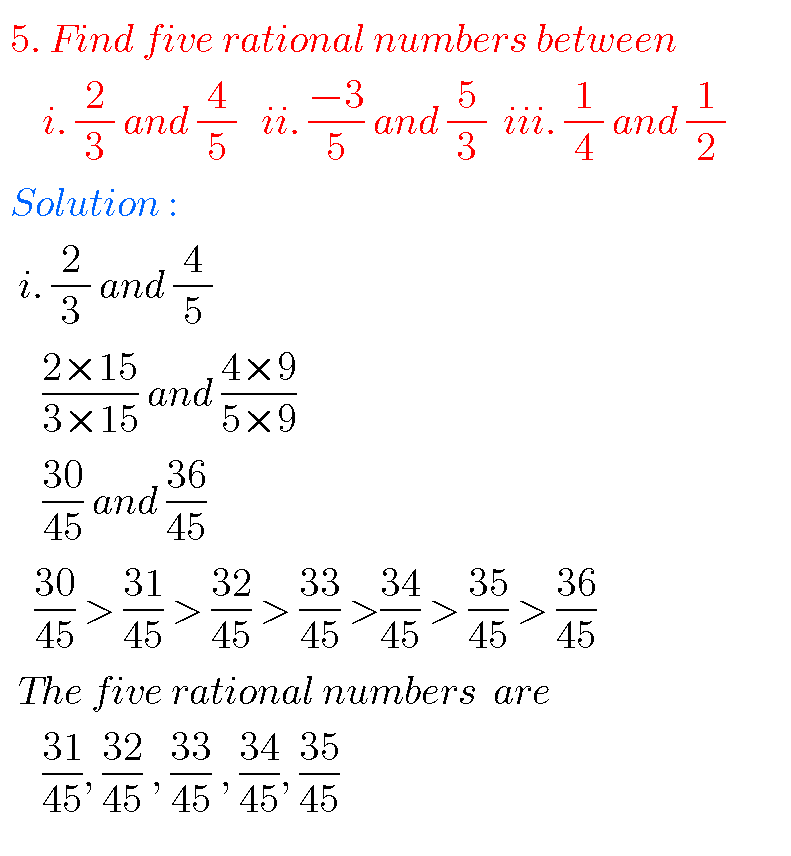# NCERT SOLUTIONS FOR CLASS 8 MATHS CHAPTER 1 RATIONAL NUMBERS

Rational Numbers chapter 1 class 8 Maths Ncert solutions are given.

First you study the textbook lesson.

Observe the example problems and solutions given in the textbook.

NCERT class 8 maths solutions

## VIII CLASS RATIONAL NUMBERS SOLUTIONS class 8 NCERT

You can see ncert solutions for some chapters for 8th class maths.

Chapter 2 linear equations in one variable

Maths solutions class 10 real numbers

### RATIONAL NUMBERS SOLUTIONS NCERT class 8

EXERCISE 1.1Some other problems and solutions.# VIII CLASS RATIONAL NUMBERS SOLUTIONS

EXERCISE 1.2  (Delated for 2023 – 2024)Note : Observe the solutions of rational numbers and try them in your own methods.

You can also see

Ncert solutions for maths class 6 some chapters

Ncert solutions for maths class 7 some chapters

Ncert solutions for maths class 8 some chapters

Intermediate first year maths some chapters

Ncert solutions for class 8 chapter 12

Nios solutions for maths 311 book 1 Sets 1.1

### 3 thoughts on “Ncert solutions for class 8 maths chapter 1,Rational Numbers solutions/class viii Rational numbers solutions.”

1.1.2.